# Differentiability of a Function and Rate of Change JEE Notes | EduRev

## JEE : Differentiability of a Function and Rate of Change JEE Notes | EduRev

The document Differentiability of a Function and Rate of Change JEE Notes | EduRev is a part of the JEE Course Mathematics (Maths) Class 12.
All you need of JEE at this link: JEE

D. Differentiability

Definition of Tangent : If f is defined on an open interval containing c, and if the limit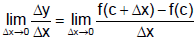= m exists, then the line passing through (c, f(c)) with slope m is the tangent line to the graph of f at the point (c, f(c)).

The slope of the tangent line to the graph of f at the point (c, f(c)) is also called the slope of the graph of f at x = c.

The above definition of a tangent line to a curve does not cover the possibility of a vertical tangent line. For vertical tangent lines, you can use the following definition. If f is continuous at c and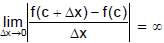then the vertical line, x = c, passing through (c, f(c)) is a vertical tangent line to the graph of f. For example, the function shown in Figure has a vertical tangent line at (c, f(c)). If the domain of f is the closed interval [a, b], then you can extend the definition of a vertical tangent line to include the endpoints by considering continuity and limits from the right (for x = a) and from the left (for x = b).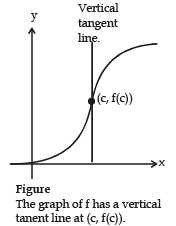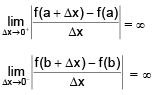In the preceding section we considered the derivative of a function f at a fixed number a :.....(1)

Note that alternatively, we can define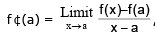provided the limit exists.

Here we change our point of view and let the number a vary. If we replace a in Equation 1 by a variable x,

we obtain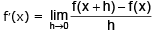...(2)

Given any number x for which this limit exists, we assign to x the number f'(x). So we can regard f' as a new function, called the derivative of f and defined by Equation 2. We know that the value of f'(x), can be interpreted geometrically as the slope of the tangent line to the graph of f at the point (x, f(x)).

The function f' is called the derivative of f because it has been "derived" from f by the limiting operation in Equation 2. The domain of f' is the set {x|f'(x) exists} and may be smaller than the domain of f.

Average And Instantaneous Rate Of Change

Suppose y is a function of x, say y = f(x). Corresponding to a change from x to x + Δx, the variable y changes from f(x) to f(x + Δx). The change in y is Δy = f(x + Δx) – f(x), and the average rate of change of y with respect to x is

Average rate of change =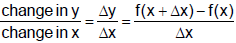As the interval over which we are averaging becomes shorter (that is, as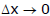), the average rate of change approaches what we would intuitively call the instantaneous rate of change of y with respect to x, and the difference quotient approaches the derivative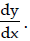Thus, we have

Instantaneous Rate of Change =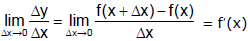To summarize :

Instantaneous Rate of Change

Suppopse f(x) is differentiable at x = x0. Then the instantaneous rate of cange of y = f(x) with respect to x at x0 is the value of the derivative of f at x0. That is

Instantaneous Rate of Change = f'(x0) =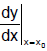Ex.13 Find the rate at which the function y = x2 sin x is changing with respect to x when x =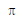.

For any x, the instantaneous rate of change in the derivative,

Sol.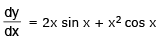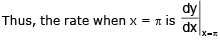= 2π sin π + π2 cos π = 2π(0) + π2 (-1) = -π2

The negative sign indicates that when x = π , the function is decreasing at the rate of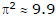units of y for each one-unit increase in x.

Let us consider an example comparing the average rate of change and the instantaneous rate of change.

Ex.14 Let f(x) = x2 - 4x + 7.

(a) Find the instantaneous rate of change of f at x = 3.

(b) Find the average rate of change of f with respect to

x between x = 3 and 5.

Sol.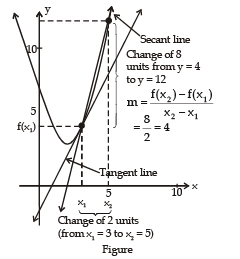(a) The derivative of the function is f'(x) = 2x – 4 Thus, the instantaneous rate of change of f at x = 3 is f'(3) = 2(3) – 4 = 2 The tangent line at x = 3 has slope 2, as shown in the figure

(b) The (average) rate of change from x = 3 to x = 5 is found by dividing the change  in f by the change in x. The change in f from x = 3 to x = 5 is

f(5) – f(3) = [52 – 4(5) + 7] – [32 – 4(3) + 7] = 8

Thus, the average rate of change is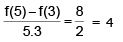The slope of the secant line is 4, as shown in the figure.

Offer running on EduRev: Apply code STAYHOME200 to get INR 200 off on our premium plan EduRev Infinity!

## Mathematics (Maths) Class 12

209 videos|222 docs|124 tests

,

,

,

,

,

,

,

,

,

,

,

,

,

,

,

,

,

,

,

,

,

;